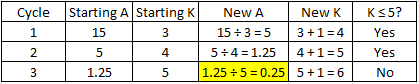## Pseudocode

The following pseudocode snippet describes a segment of a computer program. What value of A is printed?
SET A = 15
SET K = 3
While K ≤ 5
A = A/K
K = K + 1
End While
Print A

Hint
The code is running a loop. $$K$$ increases incrementally every time the code cycles through.
Hint 2
Factor in the exit criteria for the loop to end. Track the $$K$$ and $$A$$ values for each cycle.
The code is running a loop. $$K$$ increases incrementally every time the code cycles through. Although the exit criteria is based on $$K$$ , we’ll need to track both $$K$$ and $$A$$ for every cycle. Running through the script logically:Loop is exited once $$K = 6$$ . When $$K = 6$$ , $$A = 0.25$$ . Thus, the value of $$A$$ that is printed is 0.25.
0.25